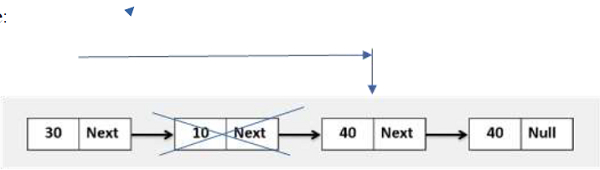# Golang program to delete the ith index node, when the index is at the mid-index level in the linked list.

## ExampleApproach to solve this problem

Step 1 − Define a method that accepts the head of a linked list.

Step 3 − When index == 0, then return head.next

Step 5 − If index i matches with the given index (to be deleted), then delete that node.next, break the loop.

Step 6 − Return at the end of the loop.

## Example

Live Demo

package main
import "fmt"
type Node struct {
value int
next *Node
}
func NewNode(value int, next *Node) *Node{
var n Node
n.value = value
n.next = next
return &n
}
for temp != nil {
fmt.Printf("%d ", temp.value)
temp = temp.next
}
fmt.Println()
}
func DeleteKthIndexNode(head *Node, index int) *Node{
}
if index == 0{
}
i := 1
for temp != nil{
if i == index{
temp.next = temp.next.next
}
i++
temp = temp.next
}
}
func main(){
head := NewNode(30, NewNode(10, NewNode(40, NewNode(40, nil))))
}
Input Linked list is: 30 10 40 40
After Deletion of 1th index node, Linked List is: 30 40 40